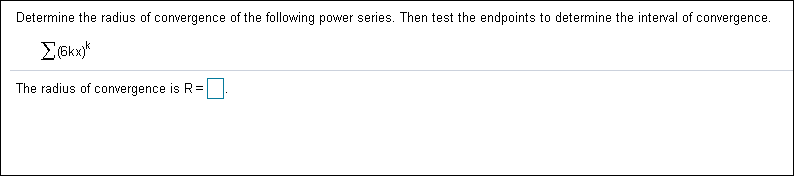# Determine the radius of convergence of the following power series. Then test the endpoints to determine the interval of convergence. Σοκ). The radius of convergence is R

Questionhelp_outlineImage TranscriptioncloseDetermine the radius of convergence of the following power series. Then test the endpoints to determine the interval of convergence. Σοκ). The radius of convergence is R fullscreen

### Want to see this answer and more?

Step-by-step answers are written by subject experts who are available 24/7. Questions are typically answered in as fast as 30 minutes.*